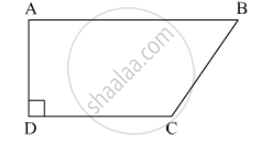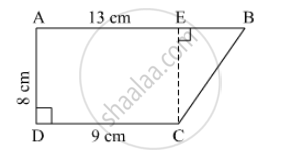SSC (English Medium) Class 8Maharashtra State Board
Share

# In ☐ Abcd, L(Ab) = 13 Cm, L(Dc) = 9 Cm, L(Ad) = 8 Cm, Find the Area of ☐ Abcd. - SSC (English Medium) Class 8 - Mathematics

ConceptIntroduction to Area of a Triangle

#### Question

In ☐ ABCD, l(AB) = 13 cm, l(DC) = 9 cm, l(AD) = 8 cm, find the area of ☐ ABCD.#### SolutionDraw perpendicular from c to line AB. Name the point E.
CE = AD = 8 cm

EB = AB - AE = AB - CD = 13 - 9 = 4cm

Area of rectangle AECD = l x b = 9 x 8 = 72 cm²

Area of Triangle BEC = 1/2 x b x h = 1/2 x 8 x 4 = 16 cm²

Area of ☐ ABCD = Area of AECD + Area of triangle BEC = 72 + 16 = 88 cm2

Is there an error in this question or solution?

#### APPEARS IN

Balbharati Solution for Balbharati Class 8 Mathematics (2019 to Current)
Chapter 15: Area
Practice Set 15.3 | Q: 1 | Page no. 99
Solution In ☐ Abcd, L(Ab) = 13 Cm, L(Dc) = 9 Cm, L(Ad) = 8 Cm, Find the Area of ☐ Abcd. Concept: Introduction to Area of a Triangle.
S